# Find overall reaction for a galvanic cell that will have the highest cell potential. What is the overall potential of the cell? Which two equations do I use?

Potential Half cell reaction
-3.05 V Li+ + e- -> Li
+1.36V Cl2 + 2e- -> 2Cl-
+2.78V F2 + 2e- -> 2F-

ReportAnswer 1

Well, you can see that all your given reactions are reductions; they reduce the oxidation state of the atom(s) to a more negative or less positive value.

(1) $\text{Li"^(+) + e^(-) -> "Li}$

${E}_{\text{red"^@ = -"3.05 V}}$

(2) ${\text{Cl"_2 + 2e^(-) -> 2"Cl}}^{-}$

${E}_{\text{red"^@ = +"1.36 V}}$

(3) ${\text{F"_2 + 2e^(-) -> 2"F}}^{-}$

${E}_{\text{red"^@ = +"2.87 V}}$ ($2.78$ is a typo)

Since one of these must be reversed to obtain an oxidation half-reaction (thereby reversing the sign of this ${E}^{\circ}$), we are looking for two half-reaction potentials that are:

• opposite in sign
• largest in magnitude overall

Thus, we choose reactions (1) and (3). To find ${E}_{\text{cell}}^{\circ}$, I prefer to use the equation

$\setminus m a t h b f \left({E}_{\text{cell"^@ = E_"red"^@ + E_"ox}}^{\circ}\right)$,

because it does not require me to think about cathodes/anodes. Instead, I just need to look at how the oxidation states changed.

We should recognize that ${E}_{\text{red"^@ = -E_"ox}}^{\circ}$ for the same half-reaction that we've reversed (the lithium one). The full reaction becomes:

${\text{F"_2(g) + cancel(2e^(-)) -> 2"F}}^{-} \left(a q\right)$
$2 \left({\text{Li"(s) -> "Li}}^{+} \left(a q\right) + \cancel{{e}^{-}}\right)$
$\text{---------------------------------------------}$
$\setminus m a t h b f \left({\text{F"_2(g) + 2"Li"(s) -> 2"Li"^(+)(aq) + 2"F}}^{-} \left(a q\right)\right)$

Note that ${E}^{\circ}$ (an intensive property) does NOT depend on the stoichiometric coefficients of the half-reaction, so you do NOT scale ${E}_{\text{ox}}^{\circ}$.

So, you just have:

color(blue)(E_"cell"^@) = E_"red"^@ + E_"ox"^@

= "2.87 V" + (-(-"3.05 V"))#

$=$ $\textcolor{b l u e}{\text{5.92 V}}$

You can see how someone else solved this problem here.

##### Add Answer of: Find overall reaction for a galvanic cell that will have the highest cell potential. What is the overall potential of the cell? Which two equations do I use?
Similar Homework Help Questions
• ### Question 7 Using the table of standard reduction potentials shown below, calculate the standard cell potential for...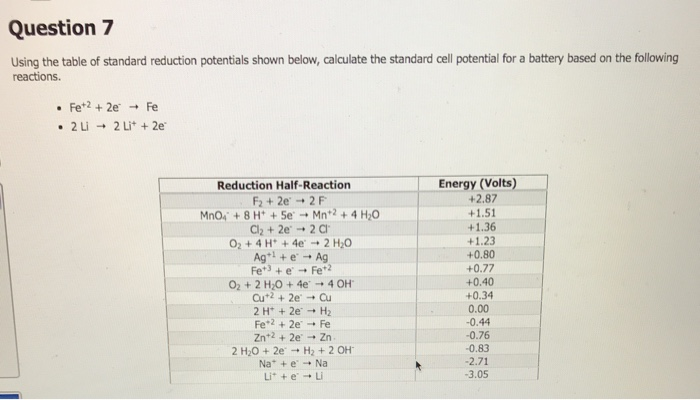Question 7 Using the table of standard reduction potentials shown below, calculate the standard cell potential for a battery based on the following reactions. • Pet2 + 2e + Fe . 2 Li + 2 Li + 2e Reduction Half-Reaction F2 +2e + 2F MnO + 8H+ Se + Mn+2+ 4 H 0 Cl2 + 2e + 20 02 + 4H' + 4e + 2 H2O Ag+ e -- Ag Fet) + e + Fe2 O2 + 2 H2O +...

• ### The overall reaction 2Co3+(aq) + 2Cl-(aq) ? 2Co2+(aq) + Cl2(g) has the standard cell voltage...

The overall reaction 2Co3+(aq) + 2Cl-(aq) ? 2Co2+(aq) + Cl2(g) has the standard cell voltage Eocell= 0.46 V.Given that Cl2(g) + 2e-? 2Cl-(aq), Eo = 1.36 V,calculate the standard reduction potential for the following the half reaction at 25oC:Co3+ + e-? Co2+1.82 V-0.90 V0.90 V-1.82 V

• ### Question 4 Using the table of standard reduction potentials shown below, calculate the standard cell potential for...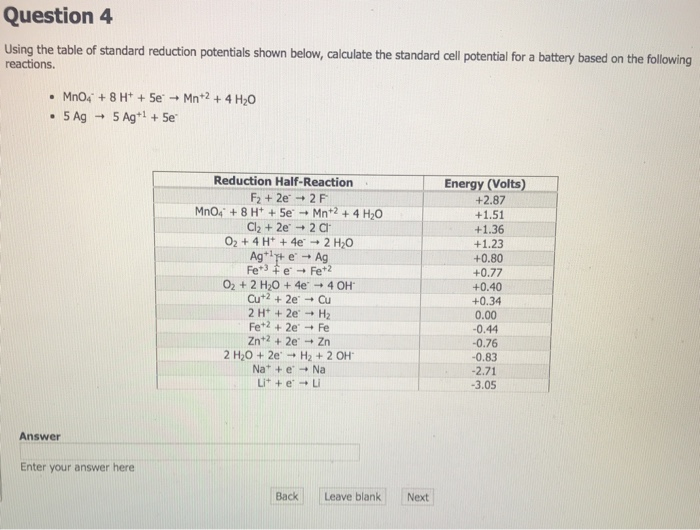Question 4 Using the table of standard reduction potentials shown below, calculate the standard cell potential for a battery based on the following reactions. • MnO4 +8H+ + 5e + Mn+2 +4 H20 . 5 Ag + 5 Ag+1 +5e Reduction Half-Reaction F2 +2e + 2F MnO4 + 8 H+ + 5e + Mn+2 + 4H2O Cl2 + 2e + 20 O2 + 4H+ + 4e + 2 H2O Ag++ e + Ag Fet3 Fe + Fet2 O2 + 2...

• ### What is the calculated value of the cell potential at 298K for an electrochemical cell with the following reaction?

What is the calculated value of the cell potential at 298K for an electrochemical cell with the following reaction, when the Cl2 pressure is 1.14 atm, the Cl- concentration is 2.62×10-3M, and the Sn2+ concentration is 7.76×10-4M ? Cl2(g) + Sn(s)=2Cl-(aq) + Sn2+(aq)

• ### use tabulated standard electrode potential to calculate the standard cell potential for the reaction occurring in an el...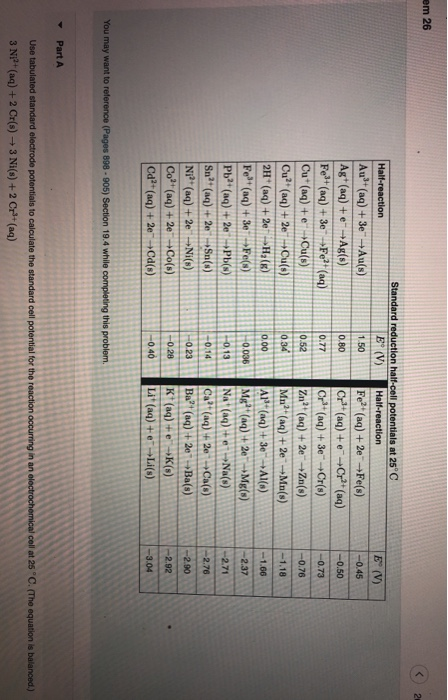use tabulated standard electrode potential to calculate the standard cell potential for the reaction occurring in an electrochemical cell at 25 C. (The equation is balanced.) 3Ni^2+(aq)+2Cr(s)--->3Ni(s)2Cr^3+(aq) Express your answer to two significant figures and include the appropriate units. em 26 E (V) -0.45 -0.50 -0.73 -0.76 -1.18 Standard reduction half-cell potentials at 25°C Half-reaction E° (V) Half-reaction Aul+ (aq) + 3e +Au(s) 1.50 Fe2+ (aq) + 2eFe(s) Ag+ (aq) +e-Ag(s) 0.80 Cr3+ (aq) + Cr²+ (aq) Fe+(aq) + 3e...

• ### Question 5 Using the table of standard reduction potentials shown below, calculate the standard cell potential for...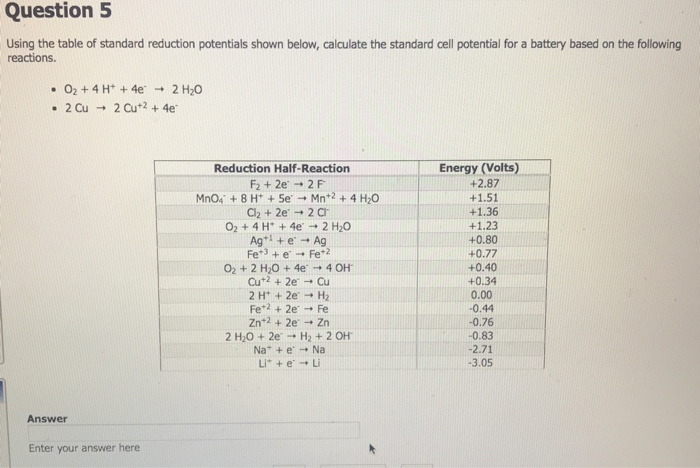Question 5 Using the table of standard reduction potentials shown below, calculate the standard cell potential for a battery based on the following reactions. • O2 + 4H+ + 4e + 2 H20 • 2 Cu + 2 Cu+2 + 4e Reduction Half-Reaction F2 + 2e + 2F MnO, +8 H+ + 5e + Mn+2 +4 H20 Cl2 + 2e → 2C O2 + 4H+ + 4e + 2 H2O Agt! + e + Ag Fet3 + e - Fet2...

• ### A chemist designs a galvanic cell that uses these two half-reactions: standard reduction potential half-reaction Br2)...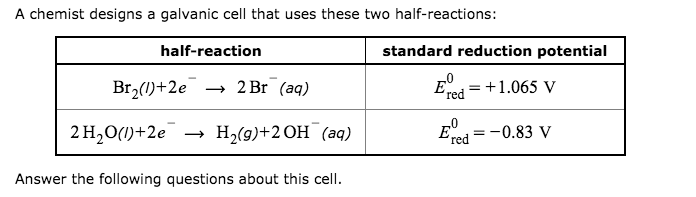A chemist designs a galvanic cell that uses these two half-reactions: standard reduction potential half-reaction Br2)+2e 2 Br (aq) 1.065 V red E 2 H,00+2е H2(9)+2ОН (ад) 0.83 V 'red Answer the following questions about this cell. Write a balanced equation for the half-reaction that happens at the cathode Write a balanced equation for the half-reaction that happens at the anode Write a balanced equation for the overall reaction that powers the cell. Be sure the reaction is spontaneous as...

• ### Write a balanced equation for the overall reaction that powers the cell. Be sure the reaction is ...Write a balanced equation for the overall reaction that powers the cell. Be sure the reaction is spontaneous as written. Do you have enough information to calculate the cell voltage under standard conditions? Yes No If you said it was possible to calculate the cell voltage, do so and enter your answerNV here. Be sure your answer has the correct number of significant digits A chemist designs a galvanic cell that uses these two half-reactions half-reaction standard reduction potential Eed+1.51...

• ### *The Nernst Equation and pH* Sulfuric acid is a very strong acid that can act as an oxidizing agent at high concentrations (low pH)

*The Nernst Equation and pH* Sulfuric acid is a very strong acid that can act as an oxidizing agent at high concentrations (low pH). Under standard conditions, sulfuric acid has a low reduction potential, SO4^2-(aq) + 4H^+(aq) + 2e^- <=> SO2(g) + 2H2O(l),.....+0.20V which means it cannot oxidize any of the halides F2, Cl2, Br2, or I2. If the H^+ ion concentration is increased, however, the driving force for the sulfuric acid reduction is also increased according to Le Châtelier's...

• ### Please show all work step by step and final answer. Reactions at Electrodes during Electrolysis Answer the following que...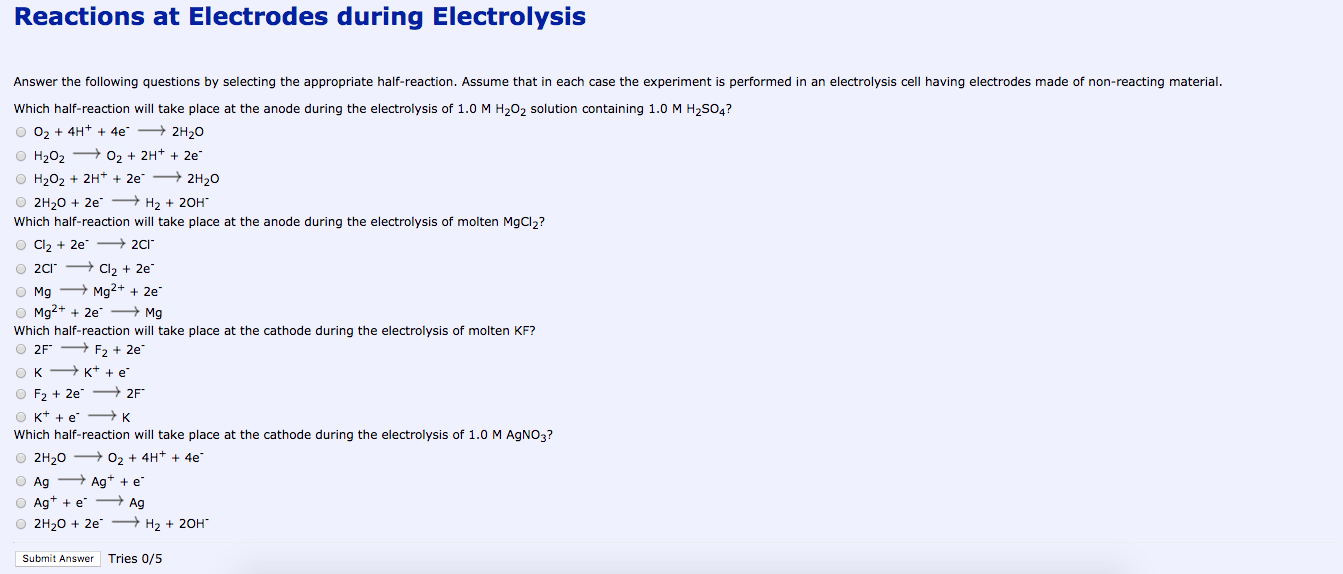Please show all work step by step and final answer. Reactions at Electrodes during Electrolysis Answer the following questions by selecting the appropriate half-reaction. Assume that in each case the experiment is performed in an electrolysis cell having electrodes made of non-reacting material. Which half-reaction will take place at the anode during the electrolysis of 1.0 M H2O2 solution containing 1.0 M H2SO4? O 02 + 4H+ + 4e - 2H20 H202 0 2 + 2H+ + 2e H2O2 +...

Need Online Homework Help?